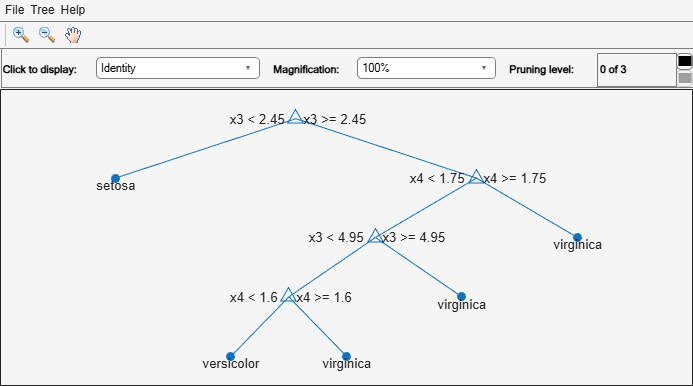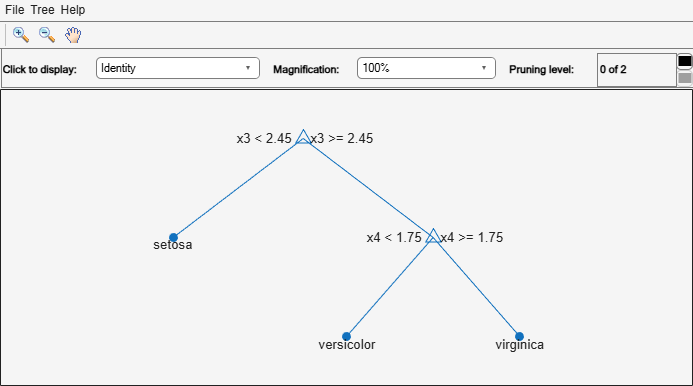# loss

Classification error

## Syntax

``L = loss(tree,TBL,ResponseVarName)``
``L = loss(tree,TBL,Y)``
``L = loss(tree,X,Y)``
``L = loss(___,Name,Value)``
``````[L,se,NLeaf,bestlevel] = loss(___)``````

## Description

````L = loss(tree,TBL,ResponseVarName)` returns a scalar representing how well `tree` classifies the data in `TBL`, when `TBL.ResponseVarName` contains the true classifications.When computing the loss, `loss` normalizes the class probabilities in `Y` to the class probabilities used for training, stored in the `Prior` property of `tree`.```
````L = loss(tree,TBL,Y)` returns a scalar representing how well `tree` classifies the data in `TBL`, when `Y` contains the true classifications.```
````L = loss(tree,X,Y)` returns a scalar representing how well `tree` classifies the data in `X`, when `Y` contains the true classifications.```
````L = loss(___,Name,Value)` returns the loss with additional options specified by one or more `Name,Value` pair arguments, using any of the previous syntaxes. For example, you can specify the loss function or observation weights.```
``````[L,se,NLeaf,bestlevel] = loss(___)``` also returns the vector of standard errors of the classification errors (`se`), the vector of numbers of leaf nodes in the trees of the pruning sequence (`NLeaf`), and the best pruning level as defined in the `TreeSize` name-value pair (`bestlevel`).```

## Input Arguments

expand all

Trained classification tree, specified as a `ClassificationTree` or `CompactClassificationTree` model object. That is, `tree` is a trained classification model returned by `fitctree` or `compact`.

Sample data, specified as a table. Each row of `TBL` corresponds to one observation, and each column corresponds to one predictor variable. Optionally, `TBL` can contain additional columns for the response variable and observation weights. `TBL` must contain all the predictors used to train `tree`. Multicolumn variables and cell arrays other than cell arrays of character vectors are not allowed.

If `TBL` contains the response variable used to train `tree`, then you do not need to specify `ResponseVarName` or `Y`.

If you train `tree` using sample data contained in a `table`, then the input data for this method must also be in a table.

Data Types: `table`

Data to classify, specified as a numeric matrix. Each row of `X` represents one observation, and each column represents one predictor. `X` must have the same number of columns as the data used to train `tree`. `X` must have the same number of rows as the number of elements in `Y`.

Data Types: `single` | `double`

Response variable name, specified as the name of a variable in `TBL`. If `TBL` contains the response variable used to train `tree`, then you do not need to specify `ResponseVarName`.

If you specify `ResponseVarName`, then you must do so as a character vector or string scalar. For example, if the response variable is stored as `TBL.Response`, then specify it as `'Response'`. Otherwise, the software treats all columns of `TBL`, including `TBL.ResponseVarName`, as predictors.

The response variable must be a categorical, character, or string array, logical or numeric vector, or cell array of character vectors. If the response variable is a character array, then each element must correspond to one row of the array.

Data Types: `char` | `string`

Class labels, specified as a categorical, character, or string array, a logical or numeric vector, or a cell array of character vectors. `Y` must be of the same type as the classification used to train `tree`, and its number of elements must equal the number of rows of `X`.

Data Types: `categorical` | `char` | `string` | `logical` | `single` | `double` | `cell`

### Name-Value Arguments

Specify optional comma-separated pairs of `Name,Value` arguments. `Name` is the argument name and `Value` is the corresponding value. `Name` must appear inside quotes. You can specify several name and value pair arguments in any order as `Name1,Value1,...,NameN,ValueN`.

Loss function, specified as the comma-separated pair consisting of `'LossFun'` and a built-in, loss-function name or function handle.

• The following table lists the available loss functions. Specify one using its corresponding character vector or string scalar.

ValueDescription
`'binodeviance'`Binomial deviance
`'classiferror'`Misclassified rate in decimal
`'exponential'`Exponential loss
`'hinge'`Hinge loss
`'logit'`Logistic loss
`'mincost'`Minimal expected misclassification cost (for classification scores that are posterior probabilities)
`'quadratic'`Quadratic loss

`'mincost'` is appropriate for classification scores that are posterior probabilities. Classification trees return posterior probabilities as classification scores by default (see `predict`).

• Specify your own function using function handle notation.

Suppose that `n` be the number of observations in `X` and `K` be the number of distinct classes (`numel(tree.ClassNames)`). Your function must have this signature

``lossvalue = lossfun(C,S,W,Cost)``
where:

• The output argument `lossvalue` is a scalar.

• You choose the function name (`lossfun`).

• `C` is an `n`-by-`K` logical matrix with rows indicating which class the corresponding observation belongs. The column order corresponds to the class order in `tree.ClassNames`.

Construct `C` by setting `C(p,q) = 1` if observation `p` is in class `q`, for each row. Set all other elements of row `p` to `0`.

• `S` is an `n`-by-`K` numeric matrix of classification scores. The column order corresponds to the class order in `tree.ClassNames`. `S` is a matrix of classification scores, similar to the output of `predict`.

• `W` is an `n`-by-1 numeric vector of observation weights. If you pass `W`, the software normalizes them to sum to `1`.

• `Cost` is a K-by-`K` numeric matrix of misclassification costs. For example, ```Cost = ones(K) - eye(K)``` specifies a cost of `0` for correct classification, and `1` for misclassification.

Specify your function using `'LossFun',@lossfun`.

For more details on loss functions, see Classification Loss.

Data Types: `char` | `string` | `function_handle`

Observation weights, specified as the comma-separated pair consisting of `'Weights'` and a numeric vector of positive values or the name of a variable in `TBL`.

If you specify `Weights` as a numeric vector, then the size of `Weights` must be equal to the number of rows in `X` or `TBL`.

If you specify `Weights` as the name of a variable in `TBL`, you must do so as a character vector or string scalar. For example, if the weights are stored as `TBL.W`, then specify it as `'W'`. Otherwise, the software treats all columns of `TBL`, including `TBL.W`, as predictors.

`loss` normalizes the weights so that observation weights in each class sum to the prior probability of that class. When you supply `Weights`, `loss` computes weighted classification loss.

Data Types: `single` | `double` | `char` | `string`

`Name,Value` arguments associated with pruning subtrees:

Pruning level, specified as the comma-separated pair consisting of `'Subtrees'` and a vector of nonnegative integers in ascending order or `'all'`.

If you specify a vector, then all elements must be at least `0` and at most `max(tree.PruneList)`. `0` indicates the full, unpruned tree and `max(tree.PruneList)` indicates the completely pruned tree (i.e., just the root node).

If you specify `'all'`, then `loss` operates on all subtrees (i.e., the entire pruning sequence). This specification is equivalent to using `0:max(tree.PruneList)`.

`loss` prunes `tree` to each level indicated in `Subtrees`, and then estimates the corresponding output arguments. The size of `Subtrees` determines the size of some output arguments.

To invoke `Subtrees`, the properties `PruneList` and `PruneAlpha` of `tree` must be nonempty. In other words, grow `tree` by setting `'Prune','on'`, or by pruning `tree` using `prune`.

Example: `'Subtrees','all'`

Data Types: `single` | `double` | `char` | `string`

Tree size, specified as the comma-separated pair consisting of `'TreeSize'` and one of the following values:

• `'se'``loss` returns the highest pruning level with loss within one standard deviation of the minimum (`L`+`se`, where `L` and `se` relate to the smallest value in `Subtrees`).

• `'min'``loss` returns the element of `Subtrees` with smallest loss, usually the smallest element of `Subtrees`.

## Output Arguments

expand all

Classification loss, returned as a vector the length of `Subtrees`. The meaning of the error depends on the values in `Weights` and `LossFun`.

Standard error of loss, returned as a vector the length of `Subtrees`.

Number of leaves (terminal nodes) in the pruned subtrees, returned as a vector the length of `Subtrees`.

Best pruning level as defined in the `TreeSize` name-value pair, returned as a scalar whose value depends on `TreeSize`:

• `TreeSize` = `'se'``loss` returns the highest pruning level with loss within one standard deviation of the minimum (`L`+`se`, where `L` and `se` relate to the smallest value in `Subtrees`).

• `TreeSize` = `'min'``loss` returns the element of `Subtrees` with smallest loss, usually the smallest element of `Subtrees`.

By default, `bestlevel` is the pruning level that gives loss within one standard deviation of minimal loss.

## Examples

expand all

Compute the resubstituted classification error for the `ionosphere` data set.

```load ionosphere tree = fitctree(X,Y); L = loss(tree,X,Y)```
```L = 0.0114 ```

Unpruned decision trees tend to overfit. One way to balance model complexity and out-of-sample performance is to prune a tree (or restrict its growth) so that in-sample and out-of-sample performance are satisfactory.

Load Fisher's iris data set. Partition the data into training (50%) and validation (50%) sets.

```load fisheriris n = size(meas,1); rng(1) % For reproducibility idxTrn = false(n,1); idxTrn(randsample(n,round(0.5*n))) = true; % Training set logical indices idxVal = idxTrn == false; % Validation set logical indices```

Grow a classification tree using the training set.

`Mdl = fitctree(meas(idxTrn,:),species(idxTrn));`

View the classification tree.

`view(Mdl,'Mode','graph');`The classification tree has four pruning levels. Level 0 is the full, unpruned tree (as displayed). Level 3 is just the root node (i.e., no splits).

Examine the training sample classification error for each subtree (or pruning level) excluding the highest level.

```m = max(Mdl.PruneList) - 1; trnLoss = resubLoss(Mdl,'SubTrees',0:m)```
```trnLoss = 3×1 0.0267 0.0533 0.3067 ```
• The full, unpruned tree misclassifies about 2.7% of the training observations.

• The tree pruned to level 1 misclassifies about 5.3% of the training observations.

• The tree pruned to level 2 (i.e., a stump) misclassifies about 30.6% of the training observations.

Examine the validation sample classification error at each level excluding the highest level.

`valLoss = loss(Mdl,meas(idxVal,:),species(idxVal),'SubTrees',0:m)`
```valLoss = 3×1 0.0369 0.0237 0.3067 ```
• The full, unpruned tree misclassifies about 3.7% of the validation observations.

• The tree pruned to level 1 misclassifies about 2.4% of the validation observations.

• The tree pruned to level 2 (i.e., a stump) misclassifies about 30.7% of the validation observations.

To balance model complexity and out-of-sample performance, consider pruning `Mdl` to level 1.

```pruneMdl = prune(Mdl,'Level',1); view(pruneMdl,'Mode','graph')```expand all